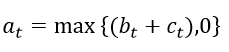# Max min function

Hi, all.
One of my equations is as follows:I linearized this equation:and wrote its dynare code in the same way.

The error that dynare pointed out to me was as follows:
WARNING: you have declared your model ‘linear’ but you are using a function (max, min, abs, sign) or an operator (<, >, <=, >=, ==, !=) which potentially makes it non-linear.

My first question is whether my linearization is correct? In fact, I did not consider the maximum in the linearization. I do not think this is correct !!!

My second question is, should I consider the model nonlinear?
Is there a similar model with dynare code available that I can use?

No, your linearization is not correct as you do not take into account the derivatives of max/min. According to the manual:

Note that these functions are differentiable everywhere except on a line of the 2-dimensional real plane defined by a=b. However for facilitating convergence of Newton-type methods, Dynare assumes that, at the points of non-differentiability, the partial derivative of these functions with respect to the first (resp. the second) argument is equal to 1 (resp. to 0) (i.e. the derivatives at the kink are equal to the derivatives observed on the half-plane where the function is equal to its first argument).

The error message you get is due to the fact that you have `model(linear)` in your mod file, which, as you are using max or min operators is not correct, because this introduces nonlinearity into your model.

Regarding examples on how to introduce max/min (or better using mcp tags and the lmmcp solver), you can have a look at our mod files in our testsuite:

Hi, @wmutschl.

Just to ask you a quick follow up question: does max/min do the exact same as `mcp` tag when it comes to solving `lmmcp`?

In effect, yes. But the model needs to be set up with the appropriate complementarity condition for the algorithm to work.

1 Like

Thanks!